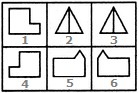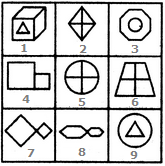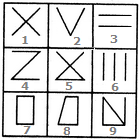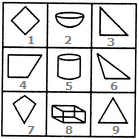# Non Verbal Reasoning - Grouping of Images

## Why Non Verbal Reasoning Grouping of Images?

In this section you can learn and practice Non Verbal Reasoning Questions based on "Grouping of Images" and improve your skills in order to face the interview, competitive examination and various entrance test (CAT, GATE, GRE, MAT, Bank Exam, Railway Exam etc.) with full confidence.

## Where can I get Non Verbal Reasoning Grouping of Images questions and answers with explanation?

IndiaBIX provides you lots of fully solved Non Verbal Reasoning (Grouping of Images) questions and answers with Explanation. Solved examples with detailed answer description, explanation are given and it would be easy to understand. All students, freshers can download Non Verbal Reasoning Grouping of Images quiz questions with answers as PDF files and eBooks.

## Where can I get Non Verbal Reasoning Grouping of Images Interview Questions and Answers (objective type, multiple choice)?

Here you can find objective type Non Verbal Reasoning Grouping of Images questions and answers for interview and entrance examination. Multiple choice and true or false type questions are also provided.

## How to solve Non Verbal Reasoning Grouping of Images problems?

You can easily solve all kind of Non Verbal Reasoning questions based on Grouping of Images by practicing the objective type exercises given below, also get shortcut methods to solve Non Verbal Reasoning Grouping of Images problems.

### Exercise :: Grouping of Images - Section 1

In each of the following questions, group the given figures into three classes using each figure only once.

1.

Group the given figures into three classes using each figure only once.A. 1,4 ; 2,3 ; 5,6 B. 1,5 ; 2,6 ; 4,3 C. 1,6 ; 2,3 ; 4,5 D. 1,2 ; 3,6 ; 4,5

Explanation:

(1, 4), (2, 3) and (5, 6) are three different pairs of identical figures.

2.

Group the given figures into three classes using each figure only once.A. 1,3,9 ; 2,5,6 ; 4,7,8 B. 1,3,9 ; 2,7,8 ; 4,5,6 C. 1,2,4 ; 3,5,7 ; 6,8,9 D. 1,3,6 ; 2,4,8 ; 5,7,9

Explanation:

1, 3, 9 have one element placed inside a different element.

2, 5, 6 contain two mutually perpendicular lines dividing the figure into four parts.

4, 7, 8 have two similar elements (unequal in size) attached to each other.

3.

Group the given figures into three classes using each figure only once.A. 7,8,9 ; 2,4,3 ; 1,5,6 B. 1,3,2 ; 4,5,7 ; 6,8,9 C. 1,6,8 ; 3,4,7 ; 2,5,9 D. 1,6,9 ; 3,4,7 ; 2,5,8

Explanation:

1, 6, 9, are all triangles.

3, 4, 7 are all four-sided figures.

2, 5, 8 are all five-sided figures.

4.

Group the given figures into three classes using each figure only once.A. 1,2,3 ; 4,5,6 ; 7,8,9 B. 1,3,5 ; 2,4,6 ; 7,8,9 C. 1,5,9 ; 3,6,2 ; 4,7,8 D. 1,9,7 ; 2,8,5 ; 3,4,6

Explanation:

1, 2, 3 are figures composed of two straight lines.

4, 5, 6 are figures composed of three straight lines.

7, 8, 9 are figures composed of four straight lines.

5.

Group the given figures into three classes using each figure only once.A. 1,4,7 ; 2,5,8 ; 3,6,9 B. 1,4,7 ; 2,5,9 ; 3,6,7 C. 1,3,4 ; 2,5,8 ; 6,7,9 D. 1,2,3 ; 4,5,6 ; 7,8,9

Explanation:

1, 4, 7 are all (two-dimensional) quadrilaterals.

2, 5, 8 are all three-dimensional figures.

3, 6, 9 are all (two-dimensional) triangles.